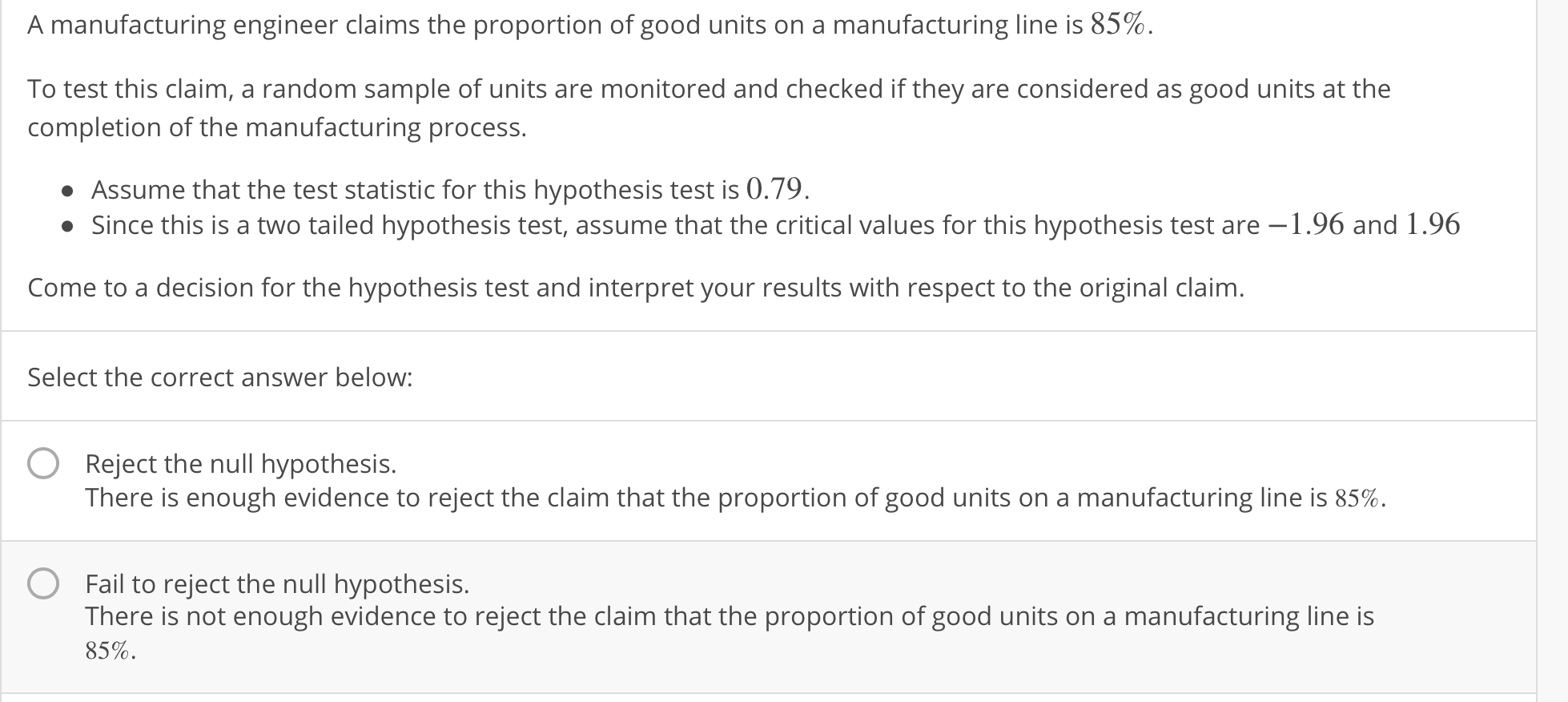A manufacturing engineer claims the proportion of good units on a manufacturing line is 85%.To test this claim, a random sample of units are monitored and checked if they are considered as good units at thecompletion of the manufacturing process.Assume that the test statistic for this hypothesis test is 0.79.Since this is a two tailed hypothesis test, assume that the critical values for this hypothesis test are -1.96 and 1.96Come to a decision for the hypothesis test and interpret your results with respect to the original claim.Select the correct answer below:OReject the null hypothesis.There is enough evidence to reject the claim that the proportion of good units on a manufacturing line is 85%.OFail to reject the null hypothesis.There is not enough evidence to reject the claim that the proportion of good units on a manufacturing line is85%.

Questionhelp_outlineImage TranscriptioncloseA manufacturing engineer claims the proportion of good units on a manufacturing line is 85%. To test this claim, a random sample of units are monitored and checked if they are considered as good units at the completion of the manufacturing process. Assume that the test statistic for this hypothesis test is 0.79. Since this is a two tailed hypothesis test, assume that the critical values for this hypothesis test are -1.96 and 1.96 Come to a decision for the hypothesis test and interpret your results with respect to the original claim. Select the correct answer below: OReject the null hypothesis. There is enough evidence to reject the claim that the proportion of good units on a manufacturing line is 85%. O Fail to reject the null hypothesis. There is not enough evidence to reject the claim that the proportion of good units on a manufacturing line is 85%. fullscreen
Step 1

Given a hypothesis test to check the proportion of good units on a manufacturing line is 85%.

In a hypothesis test if the test statistic is between the critical scores, then we do not have sufficient evidence to reject the null ...

Want to see the full answer?

See Solution

Want to see this answer and more?

Our solutions are written by experts, many with advanced degrees, and available 24/7

See Solution
Tagged in

Hypothesis Testing